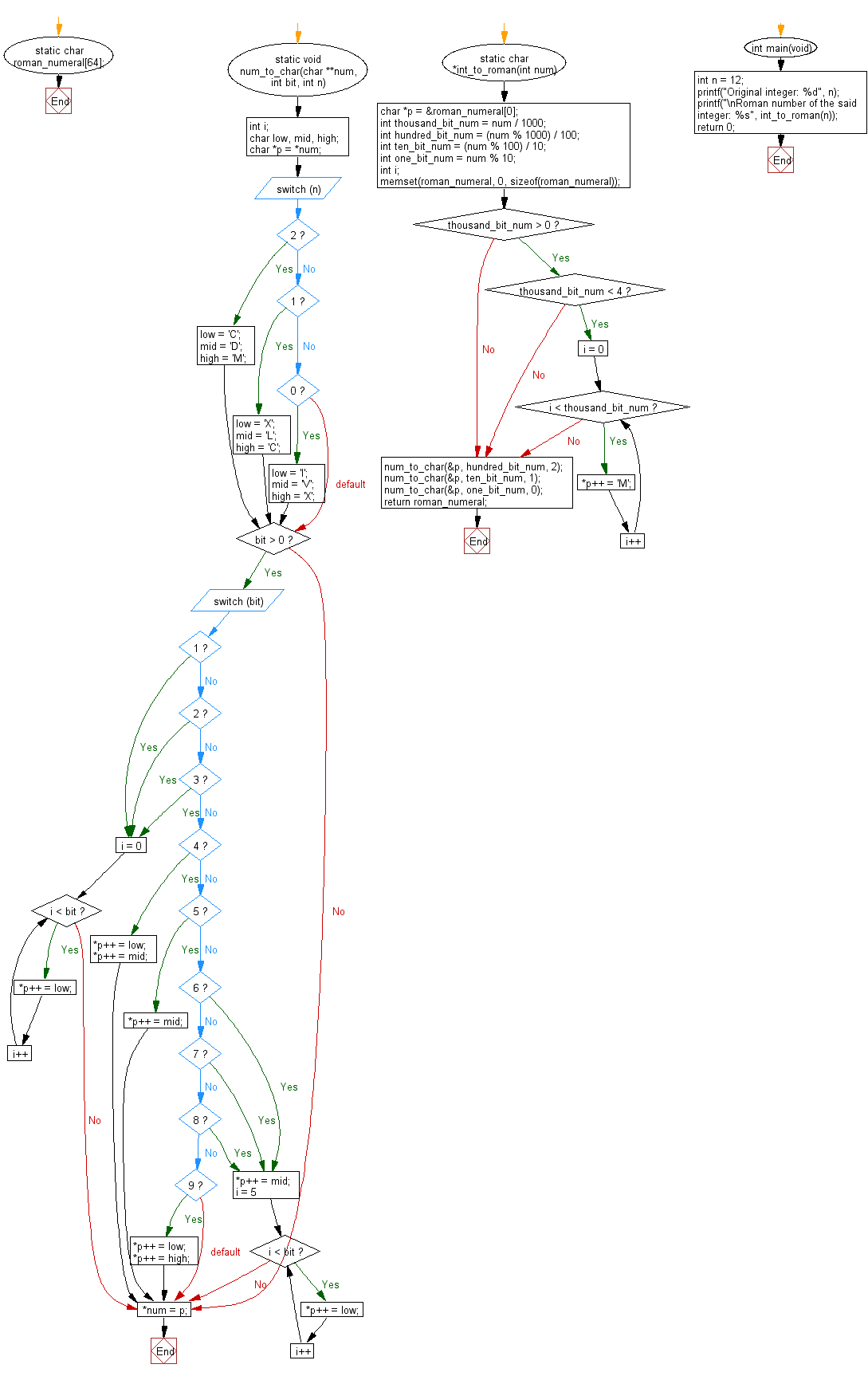﻿ C Program: Convert a given integer to roman number - w3resource# C Exercises: Convert a given integer to roman number

## C Programming Practice: Exercise-6 with Solution

Write a C programming to convert a given integer to roman number.

```Roman numerals are represented by seven different symbols: I, V, X, L, C, D and M.
Symbol       Value
I             1
V             5
X             10
L             50
C             100
D             500
M             1000
```

C Code:

``````#include <stdio.h>
#include <stdlib.h>
#include <string.h>

static void num_to_char(char **num, int bit, int n)
{
int i;
char low, mid, high;
char *p = *num;

switch (n) {
case 2:
low = 'C';
mid = 'D';
high = 'M';
break;
case 1:
low = 'X';
mid = 'L';
high = 'C';
break;
case 0:
low = 'I';
mid = 'V';
high = 'X';
break;
}

if (bit > 0) {
switch (bit) {
case 1:
case 2:
case 3:
for (i = 0; i < bit; i++) {
*p++ = low;
}
break;
case 4:
*p++ = low;
*p++ = mid;
break;
case 5:
*p++ = mid;
break;
case 6:
case 7:
case 8:
*p++ = mid;
for (i = 5; i < bit; i++) {
*p++ = low;
}
break;
case 9:
*p++ = low;
*p++ = high;
break;
}
}

*num = p;
}

static char roman_numeral;

static char *int_to_roman(int num)
{
char *p = &roman_numeral;
int thousand_bit_num = num / 1000;
int hundred_bit_num = (num % 1000) / 100;
int ten_bit_num = (num % 100) / 10;
int one_bit_num = num % 10;
int i;

memset(roman_numeral, 0, sizeof(roman_numeral));

if (thousand_bit_num > 0) {
if (thousand_bit_num < 4) {
for (i = 0; i < thousand_bit_num; i++) {
*p++ = 'M';
}
}
}

num_to_char(&p, hundred_bit_num, 2);
num_to_char(&p, ten_bit_num, 1);
num_to_char(&p, one_bit_num, 0);

return roman_numeral;
}

int main(void)
{
int n = 12;
printf("Original integer: %d", n);
printf("\nRoman number of the said integer: %s", int_to_roman(n));
return 0;
}
``````

Sample Output:

```Original integer: 12
Roman number of the said integer: XII
```

Pictorial Presentation:Flowchart:## C Programming Code Editor:

What is the difficulty level of this exercise?

Test your Programming skills with w3resource's quiz.

﻿

## C Programming: Tips of the Day

Maximum value of int:

In C:

```#include <limits.h>
then use
int imin = INT_MIN; // minimum value
int imax = INT_MAX;```

or

```#include <float.h>

float fmin = FLT_MIN;  // minimum positive value
double dmin = DBL_MIN; // minimum positive value

float fmax = FLT_MAX;
double dmax = DBL_MAX;```

Ref : https://bit.ly/3fi8yk9